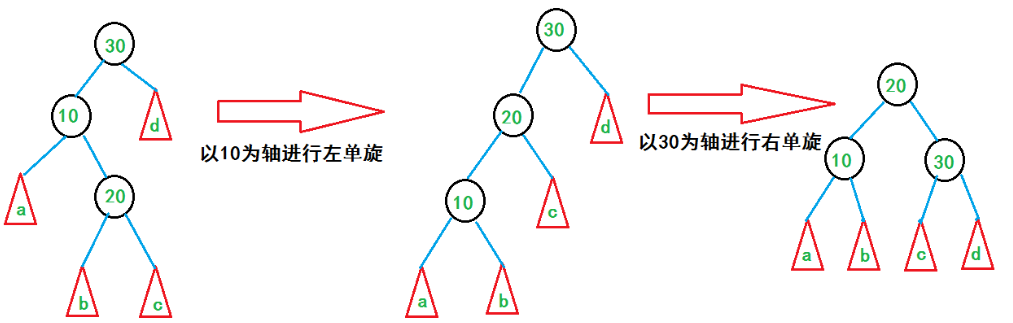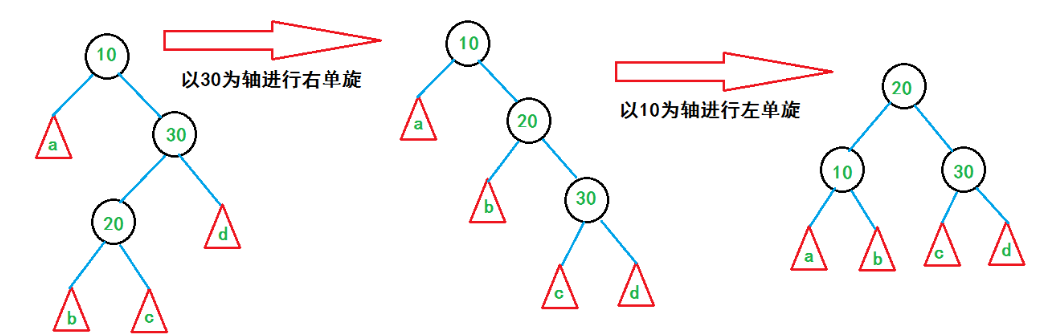8.7 平衡二叉树

# 8.7 平衡二叉树

## 什么是最小不平衡树呢？## 8.7.1 实现原理

### 1.什么是左旋，右旋，双旋## 8.7.2 平衡二叉树的实现算法

### 1.平衡二叉树的结构

``````typedef  struct BiTNode /* 结点结构 */
{
int data;   /* 结点数据 */
int bf; /*  结点的平衡因子 */
struct BiTNode *lchild, *rchild;    /* 左右孩子指针 */
} BiTNode, *BiTree;``````

### 2.右旋操作

``````    /* 对以p为根的二叉排序树作右旋处理。 */
/* 处理之后p指向新的树根结点。即旋转处理之前的左子树的根结点 */
void R_Rotate(BiTree *P)
{
BiTree L;
L=(*P)->lchild;         /*L指向P的左子树根结点 */
(*P)->lchild=L->rchild; /*L的右子树挂接为P的左子树 */
L->rchild=(*P);
*P=L; /*P指向新的根结点 */
}``````### 3.左平衡旋转代码

``````#define LH +1 /*  左高 */
#define EH 0  /*  等高 */
#define RH -1 /*  右高 */

/*  对以指针T所指结点为根的二叉树作左平衡旋转处理 */
/*  本算法结束时，指针T指向新的根结点*/
void LeftBalance(BiTree *T)
{
BiTree L,Lr;
L=(*T)->lchild; /*  L指向T的左子树根结点 */
switch(L->bf)
{ /*  检查T的左子树的平衡度，并作对应平衡处理 */
case LH: /*  新结点插入在T的左孩子的左子树上。要作单右旋处理 */
(*T)->bf=L->bf=EH;
R_Rotate(T);
break;
case RH: /*  新结点插入在T的左孩子的右子树上。要作双旋处理 */
Lr=L->rchild; /*  Lr指向T的左孩子的右子树根 */
switch(Lr->bf)
{ /*  改动T及其左孩子的平衡因子 */
case LH: (*T)->bf=RH;
L->bf=EH;
break;
case EH: (*T)->bf=L->bf=EH;
break;
case RH: (*T)->bf=EH;
L->bf=LH;
break;
}
Lr->bf=EH;
L_Rotate(&(*T)->lchild); /*  对T的左子树作左旋平衡处理 */
R_Rotate(T); /*  对T作右旋平衡处理 */
}
}``````

### 4.主函数代码

``````/*  若在平衡的二叉排序树T中不存在和e有同样关键字的结点，则插入一个 */
/*  数据元素为e的新结点。并返回1，否则返回0。若因插入而使二叉排序树 */
/*  失去平衡，则作平衡旋转处理。布尔变量taller反映T长高与否。 */
Status InsertAVL(BiTree *T,int e,Status *taller)
{
if(!*T)
{ /*  插入新结点。树“长高”，置taller为TRUE */
*T=(BiTree)malloc(sizeof(BiTNode));
(*T)->data=e; (*T)->lchild=(*T)->rchild=NULL; (*T)->bf=EH;
*taller=TRUE;
}
else
{
if (e==(*T)->data)
{ /*  树中已存在和e有同样关键字的结点则不再插入 */
*taller=FALSE; return FALSE;
}
if (e<(*T)->data)
{ /*  应继续在T的左子树中进行搜索 */
if(!InsertAVL(&(*T)->lchild,e,taller)) /*  未插入 */
return FALSE;
if(taller) /*   已插入到T的左子树中且左子树“长高” */
switch((*T)->bf) /*  检查T的平衡度 */
{
case LH: /*  原本左子树比右子树高。须要作左平衡处理 */
LeftBalance(T); *taller=FALSE; break;
case EH: /*  原本左、右子树等高，现因左子树增高而使树增高 */
(*T)->bf=LH; *taller=TRUE; break;
case RH: /*  原本右子树比左子树高，现左、右子树等高 */
(*T)->bf=EH; *taller=FALSE; break;
}
}
else
{ /*  应继续在T的右子树中进行搜索 */
if(!InsertAVL(&(*T)->rchild,e,taller)) /*  未插入 */
return FALSE;
if(*taller) /*  已插入到T的右子树且右子树“长高” */
switch((*T)->bf) /*  检查T的平衡度 */
{
case LH: /*  原本左子树比右子树高。现左、右子树等高 */
(*T)->bf=EH; *taller=FALSE; break;
case EH: /*  原本左、右子树等高，现因右子树增高而使树增高  */
(*T)->bf=RH; *taller=TRUE; break;
case RH: /*  原本右子树比左子树高，须要作右平衡处理 */
RightBalance(T); *taller=FALSE; break;
}
}
}
return TRUE;
}``````

目录